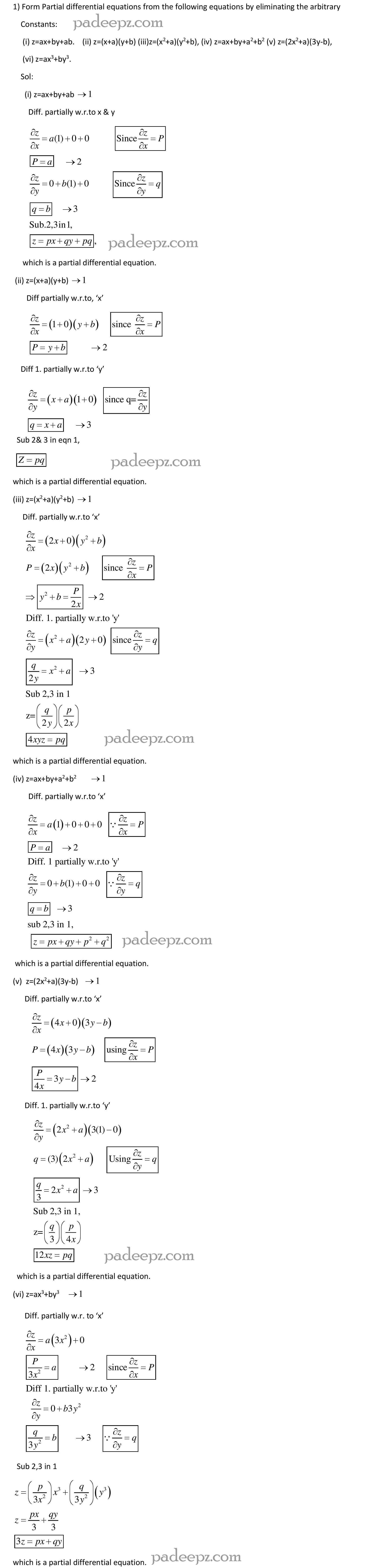MA8353 Notes Transforms and Partial Differential Equations

MA8353 Notes Transforms and Partial Differential Equations Regulation 2017 Anna University free download. Transforms and Partial Differential Equations Notes MA8353 pdf free download.

OBJECTIVES : MA8353 Notes Transforms and Partial Differential Equations

To introduce the basic concepts of PDE for solving standard partial differential equations.

To introduce Fourier series analysis which is central to many applications in engineering apart from its use in solving boundary value problems.

To acquaint the student with Fourier series techniques in solving heat flow problems used in various situations.

To acquaint the student with Fourier transform techniques used in wide variety of situations.

To introduce the effective mathematical tools for the solutions of partial differential equations that model several physical processes and to develop Z transform techniques for discrete time systems.

OUTCOMES : MA8353 Notes Transforms and Partial Differential Equations

Upon successful completion of the course, students should be able to:

Understand how to solve the given standard partial differential equations.

Solve differential equations using Fourier series analysis which plays a vital role in engineering applications.

Appreciate the physical significance of Fourier series techniques in solving one and two dimensional heat flow problems and one dimensional wave equations.

Understand the mathematical principles on transforms and partial differential equations would provide them the ability to formulate and solve some of the physical problems of engineering.

Use the effective mathematical tools for the solutions of partial differential equations by using Z transform techniques for discrete time systems.

TEXT BOOKS: MA8353 Notes Transforms and Partial Differential Equations

1. Grewal B.S., “Higher Engineering Mathematics”, 43rd Edition, Khanna Publishers, New Delhi, 2014.

2. Narayanan S., Manicavachagom Pillay.T.K and Ramanaiah.G “Advanced Mathematics for Engineering Students”, Vol. II & III, S.Viswanathan Publishers Pvt. Ltd, Chennai, 1998.

REFERENCES : MA8353 Notes Transforms and Partial Differential Equations

1. Andrews, L.C and Shivamoggi, B, “Integral Transforms for Engineers” SPIE Press, 1999.

2. Bali. N.P and Manish Goyal, “A Textbook of Engineering Mathematics”, 9th Edition, Laxmi Publications Pvt. Ltd, 2014.

3. Erwin Kreyszig, “Advanced Engineering Mathematics “, 10th Edition, John Wiley, India, 2016.

4. James, G., “Advanced Modern Engineering Mathematics”, 3rd Edition, Pearson Education, 2007.

5. Ramana. B.V., “Higher Engineering Mathematics”, McGraw Hill Education Pvt. Ltd, New Delhi, 2016.

6. Wylie, R.C. and Barrett, L.C., “Advanced Engineering Mathematics “Tata McGraw Hill Education Pvt. Ltd, 6th Edition, New Delhi, 2012.

Padeepz E-Learning Materials MA8353 Transforms and Partial Differential Equations

Padeepz E-Learning Materials for MA8353 Transforms and Partial Differential Equations we have provided the sample materials in this page.  If you like the sample and want to buy the full subject the procedure is also provided in this page.Hi Padeepz.com is a place where we make Engineering Students to understand their subjects in an easy and in a effective way. Which leads to greater knowledge in the subject and Provide plenty of time spending in innovative ideas.

(MA8353 Notes Transforms and Partial Differential Equations)

 Subject name Transforms and Partial Differential Equations Semester 3 Subject Code MA8353 Regulation 2017 regulation

MA8353 Syllabus Transforms and Partial Differential Equations

MA8353 Important questions Transforms and Partial Differential Equations

MA8353 Questions Bank Transforms and Partial Differential Equations

2 Replies to “MA8353 Notes Transforms and Partial Differential Equations Regulation 2017 Anna University”

1.Praveenkumar says:

It’s very useful

2.Praveenkumar says:

This pdf is very useful for studying